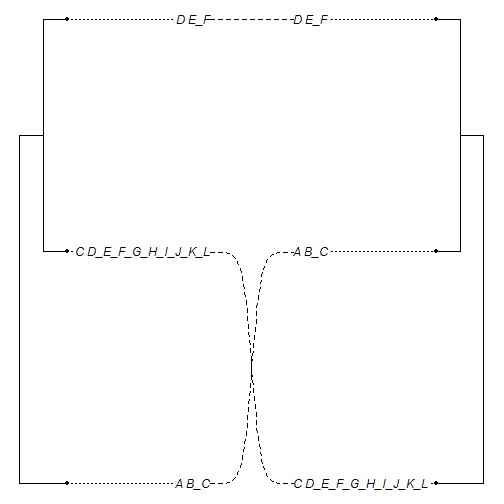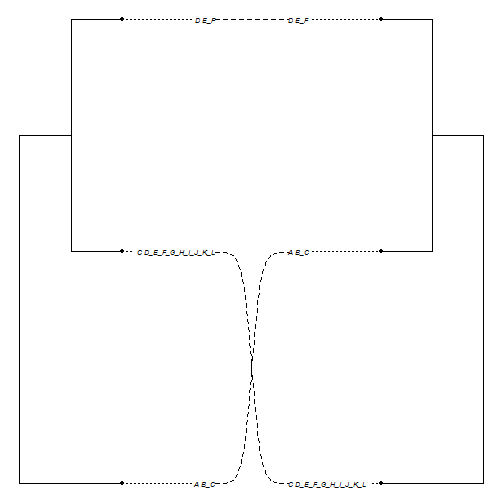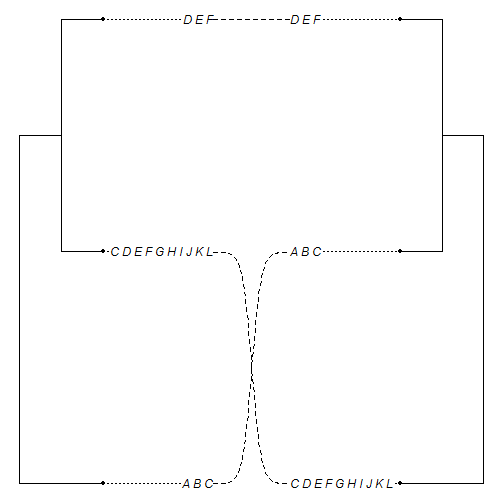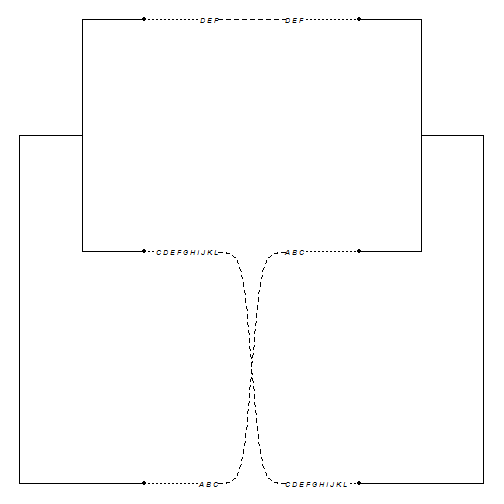## Friday, August 26, 2016

### Updates to tip label printing and `summary` method for `"cophylo"` objects

I just pushed a couple of small updates to the object class `"cophylo"` plotting method - in particular pertaining to the spacing of tip labels and what to do with underscores in species label names.

First, I recently discovered that (accidentally) by using `sub` instead of `gsub`, I was substituting only the first underscore character `"_"` for a space character `" "` in species names, instead of all such characters.

This was very easy to fix as `gsub` works the same way as `sub`, except that it substitutes all instances of a character pattern as desired, instead of just the first encountered.

Next, however, I realized that the width in a plotting device of `"_"` and `" "` differ by more than a factor of two (although this may vary between device types). E.g.:

``````plot.window(xlim=c(0,1),ylim=c(0,1))
plot.new()
````````````strwidth("_")
``````
``````##  0.02792554
``````
``````strwidth(" ")
``````
``````##  0.01196809
``````

but in computing where to draw the links to tip labels, I had been using `strwidth(tree\$tip.label)` rather than `strwidth(gsub("_"," ",tree\$tip.label))`.

Finally, I have made some changes to the S3 `summary` method for the object class `"cophylo"` so that the association matrix prints more nicely! In particular, I tried to space the columns by the longest name in the matrix, rather than just by using tabs.

So, for instance, here is the old methods:

``````library(phytools)
obj<-cophylo(t1,t2)
``````
``````## Rotating nodes to optimize matching...
## Done.
``````
``````summary(obj)
``````
``````##
## Co-phylogenetic ("cophylo") object: obj
##
## Tree 1 (left tree) is an object of class "phylo" containing 3 species.
##
## Tree 2 (right tree) is an object of class "phylo" containing 3 species.
##
## Association (assoc) table as follows:
##
##  left:   ----    right:
##   A_B_C  ----     A_B_C
##   C_D_E_F_G_H_I_J_K_L    ----     C_D_E_F_G_H_I_J_K_L
##   D_E_F  ----     D_E_F
``````
``````plot(obj,link.type="curved")
``````This problem of spacing is particularly apparent, I think, if we shrink the font size:

``````plot(obj,link.type="curved",fsize=0.6)
``````Now, let's load the updated source code from GitHub:

``````source("https://raw.githubusercontent.com/liamrevell/phytools/59f2e02a83f9142d480ad49f7e226ca0d28aeeb6/R/cophylo.R")
summary(obj)
``````
``````##
## Co-phylogenetic ("cophylo") object: obj
##
## Tree 1 (left tree) is an object of class "phylo" containing 3 species.
##
## Tree 2 (right tree) is an object of class "phylo" containing 3 species.
##
## Association (assoc) table as follows:
##
##  left:               ----    right:
##  A_B_C               ----    A_B_C
##  C_D_E_F_G_H_I_J_K_L ----    C_D_E_F_G_H_I_J_K_L
##  D_E_F               ----    D_E_F
``````
``````plot(obj,link.type="curved")
````````````plot(obj,link.type="curved",fsize=0.6)
``````Much better. phytools can also be updated to its latest version from GitHub using devtools.